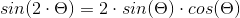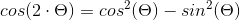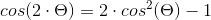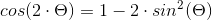# Double Angle Formula Calculator

Created by Hanna Pamuła, PhD candidate
Reviewed by Bogna Szyk and Jack Bowater
Last updated: Sep 25, 2020

Our double angle formula calculator is useful if you'd like to find all of the basic double angle identities in one place, and calculate them quickly. Such identities are useful for proving, simplifying, and solving more complicated trigonometric problems, so it's crucial that you understand and remember them. Don't worry, we'll give you a hand with that!

Whether you're searching for the sin double angle formula, or you'd love to know the derivation of the cos double angles formula, we've got you covered. Keep reading this double angle calculator, and - hopefully - trigonometric identities for double angles won't be your pain in the neck anymore.

## What is a double angle? Double angles identities

In this paragraph, we'll show you the double angles identities for sine, cosine, and tangent. To avoid misunderstandings, let's clarify at the beginning what a double angle is:

Double angle means that we increase the given angle by two

So, for example:

• 90° is the double angle for 45°
• if your given angle equals -π/3, then the double angle is -2π/3

Now we can proceed with the basic double angles identities:
1. Sin double angle formula

To calculate the double angle (2θ) of sine in terms of the original angle (θ), use the formula:You can derive this formula from the angle sum identity. As the sum of two sines is:

sin(x + y) = sin(x)*cos(y) + cos(y)*sin(x),

for a double angle we can write it as:

sin(2θ) = sin(θ + θ) = sin(θ)*cos(θ) + cos(θ)*sin(θ),

Which gives us:

sin(2θ) = 2 * sin(θ) * cos(θ)

1. Cos double angle formula

There are a few formulas for the cos double angle. The three most popular cosine of a double angle equations are:Analogically to the sine double angles identities, you can derive the first equation from the angle sum and difference identities:

cos(x + y) = cos(x)*cos(y) - sin(y)*sin(x)

For a double angle, it can be expressed as

cos(2θ) = cos(θ + θ) = cos(θ)*cos(θ) - sin(θ)*sin(θ),

therefore

cos(2θ) = cos²(θ) - sin²(θ)

To find the other two forms, use the well-known Pythagorean trigonometric identity:

sin²(θ) + cos²(θ) = 1

• Replace sin²(θ) by 1 - cos²(θ) to get the second equation:

cos(2θ) = cos²(θ) - sin²(θ) = cos²(θ) - (1 - cos²(θ)) =

2 * cos²(θ) - 1

• Replace cos²(θ) by 1 - sin²(θ) to get the third formula:

cos(2θ) = cos²(θ) - sin²(θ) = (1 - sin²(θ)) - sin²(θ) =

 1 - 2 * sin²(θ)

1. Tan double angle formula

The formula for the tangent of a double angle looks as follows:Similarly, use the sum of tangents formula:

tan(x + y) = (tan(x) + tan(y)) / 1 - tan(y)*tan(x)

For a double angle, the equation is then:

tan(2θ) = tan(θ + θ) = (tan(θ) + tan(θ)) / 1 - tan(θ) * tan(θ)

At last, we've found the final equation:

tan(2θ) = 2 * tan(θ) / (1 - tan²(θ))

## Double angles formula calculator - how to use

After all of that, are you wondering how to use this double angle calculator? The recommendation is simple - play with it! It won't explode, we promise.

However, if that advice isn't sufficient, here's a short set of instruction:

1. Decide on the units of the angle. For example, assume we would like to find out what's the double angle sine for an angle with π radians. Choose that unit from the drop-down list (π rad).
2. Input the angle's value. Let's use π/12 as an example. In that case, input 1/12 into the angle box.
3. Decide whether you'd like to see a step-by-step solution or not. By default it's shown, but you can hide it at any time.
4. That's it, the double angle formula calculator has already done the job and found the double angles of sine, cosine, and tangent. For θ = π/12, the double angle trigonometric functions look like this:
• sin(2θ) = 1/2
• cos(2θ) = √3/2
• tan(2θ) = √3/3

Additionally, the double angle formula calculator shows the equivalent of the chosen angle in degrees: π/12 = 15°

However, if you don't care much about the step-by-step solutions, you can simply use our trigonometric functions calculator - just input double the angle you're interested in directly (so for the example above, enter π/6 or 30°).

Hanna Pamuła, PhD candidate
Angle θ
deg
Step by step solution?
Double sine
Double cosine
Double tangent
People also viewed…

### Area of a hemisphere

Use this surface area of a hemisphere calculator and answer the questions: How to find the surface area of a hemisphere? What is the area of a hemisphere formula?

### Books vs e-Books

The books vs. e-books calculator answers the question: how ecological is your e-book reader? 📚

### Column space

The column space calculator will quickly give you the dimension and generators of the column space corresponding to a given matrix of size up to 4x4.

### Significant figures

The significant figures calculator performs operations on sig figs and shows you a step-by-step solution!